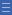## Featured resource

Home > Topdrawer > Mental computation > Activities > Facts within facts

# Facts within facts

Facts within facts

In this activity, students draw on their knowledge of known facts, doubles and partitioning to identify related facts within facts.

Using knowledge of doubles and partitioning arrays can lead to the building up of related multiplication facts.

It can also lead students to generalise that halving one factor and doubling the other gives another related fact for the product (e.g. 8 $$\times$$ 3, 4 $$\times$$ 6).

The exception is when one factor is half the other factor (e.g., 8 $$\times$$ 4, 4 $$\times$$ 8), in which case it shows the commutative fact.

You will need a blank 10 by 10 grid and a blank multiplication fact grid.

Pose the following scenario to students:

If I know 2 fours are 8, I can also work out 4 fours, 8 fours, 8 eights. What strategy might I be using?

Show 8 fours on a grid and ask students what other facts they see within this array. This brings out commutativity and the distributive property.

The following slide show outlines the activity in more detail, including further ideas and tips. Invite students to record the known fact from this on the multiplication grid.

Yes

Yes

Name Class SectionYear 3: Recall multiplication facts of two, three, five and ten and related division facts Infobox 3Year 4: Recall multiplication facts up to 10 x 10 and related division facts Infobox 3